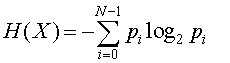# Bits of Entropy

Shannon entropy was introduced by Claude E. Shannon in the 1948 paper "A Mathematical Theory of Communication". It's this equation:Shannon entropy is the "average minimum number of bits needed to encode a string of symbols, based on the frequency of the symbols" (source).

Imagine that you and your friends have decided to communicate in an emoji-only protocol. Each emoji represents a message. But some emoji are more commonly used than others – ex. 😀  appears more in messages among your friends than 🐭. Another way of saying that is that has a higher probability of appearing in a given message than 🐭.

Since 😀   is more commonly used than 🐭, it makes sense to be smarter about encoding your messages such that 😀 takes up less space than 🐭. What Shannon entropy calculates is the average minimum number of bits needed to encode a message, since we want to send our messages in the least amount of space possible, because yay, then we can send more messages and/or be efficient and stuff!

So in this Shannon entropy calculator, our symbols are emoji, and each member of the set has a different probability of being represented in a given message. As you change the probabilities, make sure they add up to 1 (100%) since otherwise your probability distribution is incomplete.

You have 5 emoji to start with, and you can add and remove emoji to see how the number of items in the set impacts the entropy!